Next: 4.4 Discrete Cepstrum Spectral Up: 4. Estimation of Spectral Previous: 4.2 LPC Spectral Envelope

Subsections

# 4.3 Cepstrum Spectral Envelope

The cepstrum is a method of speech analysis based on a spectral representation of the signal. To explain the general idea of the cepstrum method used for spectral envelope estimation, two approaches are possible. First, one can simply think of obtaining the spectral envelope from a Fourier magnitude spectrum by successively smoothing its curve to get rid of the rapid fluctuations. This boils down to applying a low pass filter to the spectrum, interpreted as a signal, which lets only the slow fluctuations (low frequency oscillations of the curve) pass, hence the smoothing.

Second, remembering that a signal can be viewed as a convolution of a source signal with a filter, we have to somehow separate the source spectrum from the filter transfer function, which is a very good estimation of the spectral envelope.

According to the source-filter model of speech production introduced in section 2.4, a speech signal x(n) can be expressed as a convolution between a source or excitation signal e(n), produced by the glottis, and the impulse response of the vocal tract filter h(n):

x(n) = e(n) * h(n)

In frequency-domain, this convolution becomes the multiplication of the respective Fourier transforms: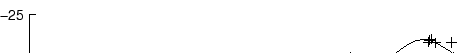Taking the logarithm of the absolute value of the Fourier transforms (the magnitude spectra, see equation (2.11)), the multiplication of equation (3.5) is converted to an addition: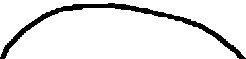If we now apply a Fourier transform 4.1 to the logarithm of the magnitude spectrum, we get the frequency distribution of the fluctuations in the curve of the spectrum c, which is called the cepstrum :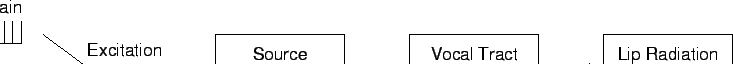Under the reasonable assumption that the source spectrum has only rapid fluctuations (the excitation signal e is a stable, regular oscillation of around 100 Hz), its contribution to c will be concentrated in its higher regions, while the contribution of H will be the slow fluctuations in the spectrum of X, and will therefore be concentrated only in the lower part of c, as can be seen in figure 3.4. Thus, the separation of the two components becomes trivial: Only the first p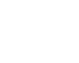of the cepstral coefficients ci are kept, where p is called the order  of the cepstrum. These represent the low frequency components, i.e. the slowly changing fluctuations, whence the smoothing of the spectrum X to become a spectral envelope. This smoothing effect can be seen in figure 3.5.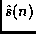tex2html_comment_mark>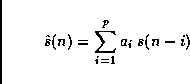tex2html_comment_mark>

The unit of the cepstrum was baptised quefrency , by virtue of inversing the syllables of frequency, analog to cepstrum stemming from an inversion of spectrum, to reflect the properties of the method. Various other nomenclatura have been invented adhering to the same style, but only these two new words have caught on.

In the taxonomy of signal processing methods, the cepstrum belongs to the class of homomorphic deconvolution  methods.

To finally obtain the spectral envelope from the cepstral coefficients, one defines the frequencies fi at which the value of the envelope is to be obtained (the bins of the envelope). Usually, one wants nequidistant frequencies up to the Nyquist frequency fs/2: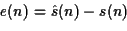Then, after passing to angular frequencies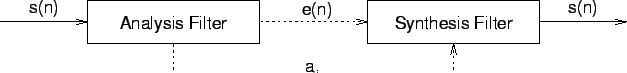the envelope value vi for frequency fi is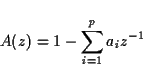Note that most of this expression is independent of the ci, especially the expensive cosine evaluation, and can therefore be precomputed as an (n, p) matrix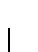with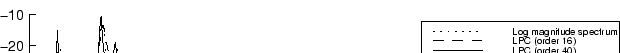so that equation (3.10) becomes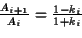#### Disadvantages of the Cepstrum Method

There are two disadvantages of the cepstrum method of spectral envelope estimation. First, as the cepstrum is essentially a low pass filtering of the curve of the spectrum interpreted as a signal, it will actually average-out the fluctuations of the curve of the spectrum. The effect can be seen in figure 3.5. This is not what we want, because then the resulting curve has no longer the enveloping property to link the peaks of the curve (cf. section 2.3).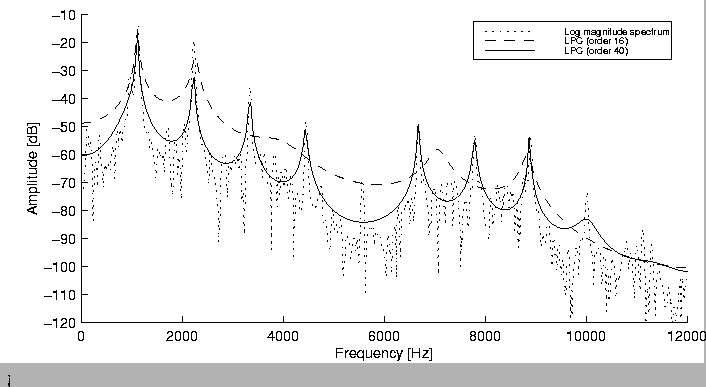tex2html_comment_mark>

Second, similar to LPC, in analysing harmonic sounds (with a conspicuous partial structure) they will follow the curve of the spectrum down to the residual noise level in the gap between two partials, especially when the partials are spaced far apart as for high pitched sounds. See figure 3.6 for an example of this behaviour.Next: 4.4 Discrete Cepstrum Spectral Up: 4. Estimation of Spectral Previous: 4.2 LPC Spectral Envelope
Diemo Schwarz
1998-09-07